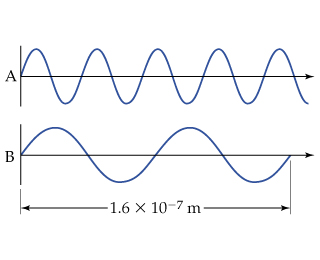# Problem: Consider the two waves shown here, which we will consider to represent two electromagnetic radiations.What is the wavelength of wave A?

###### FREE Expert Solution

We are asked to determine the wavelength of wave A in the figure:Recall that a wavelength is the distance wherein a wave repeats. Let us assign the wavelengths of the waves given:

93% (63 ratings)###### Problem Details

Consider the two waves shown here, which we will consider to represent two electromagnetic radiations.

What is the wavelength of wave A?